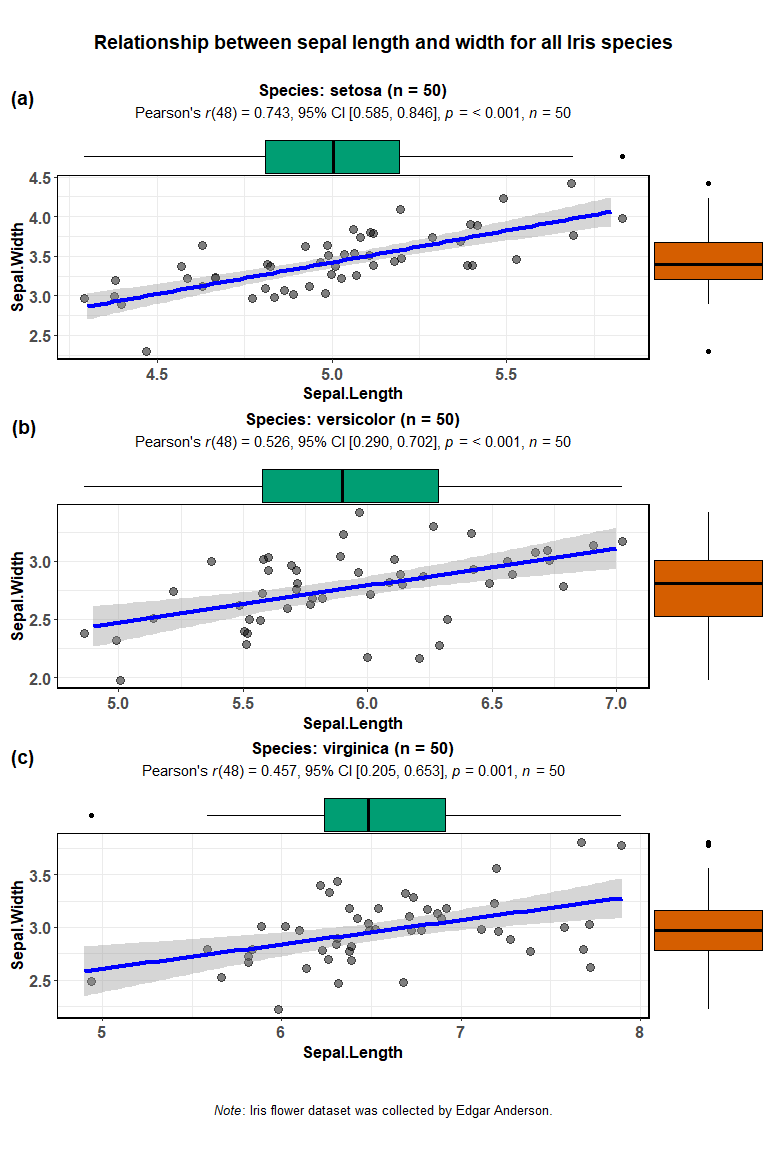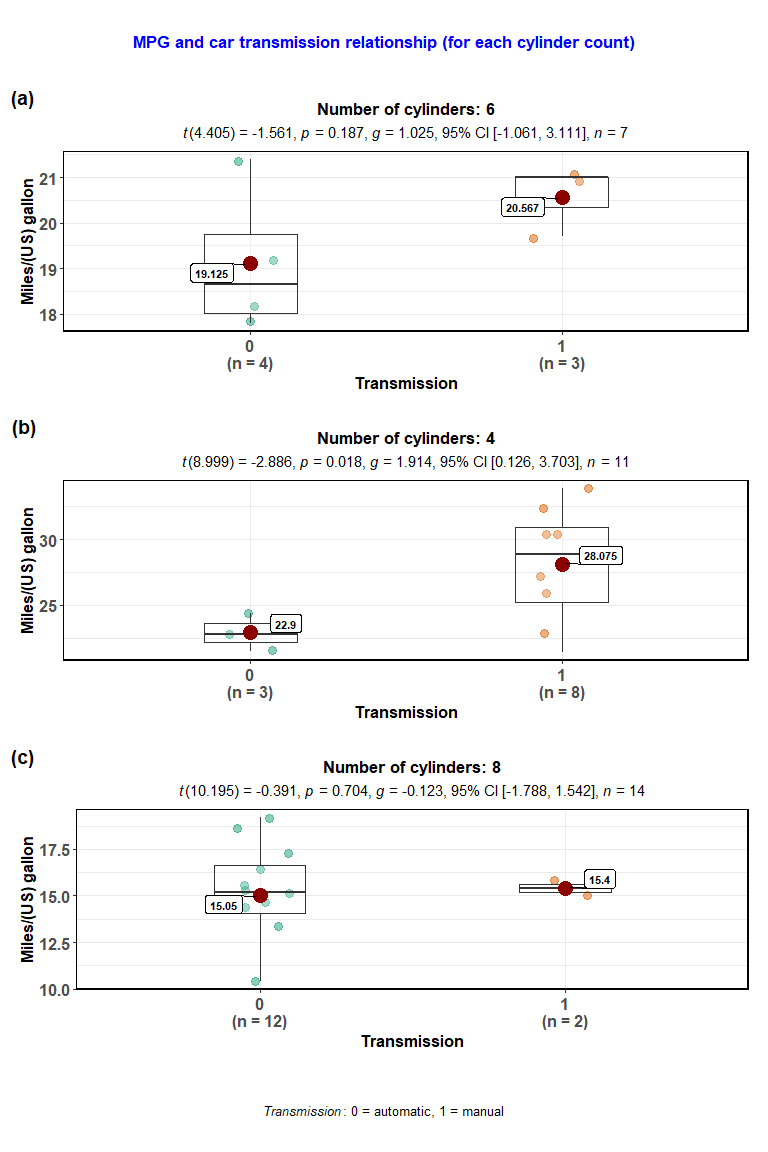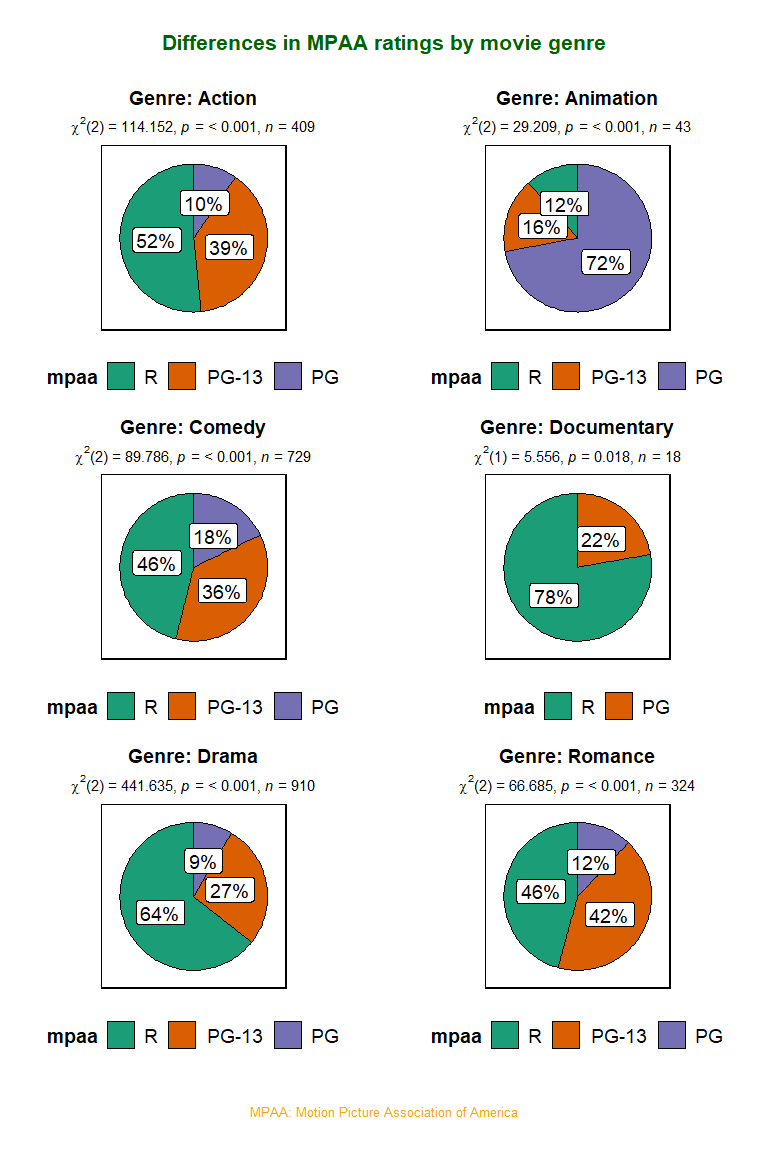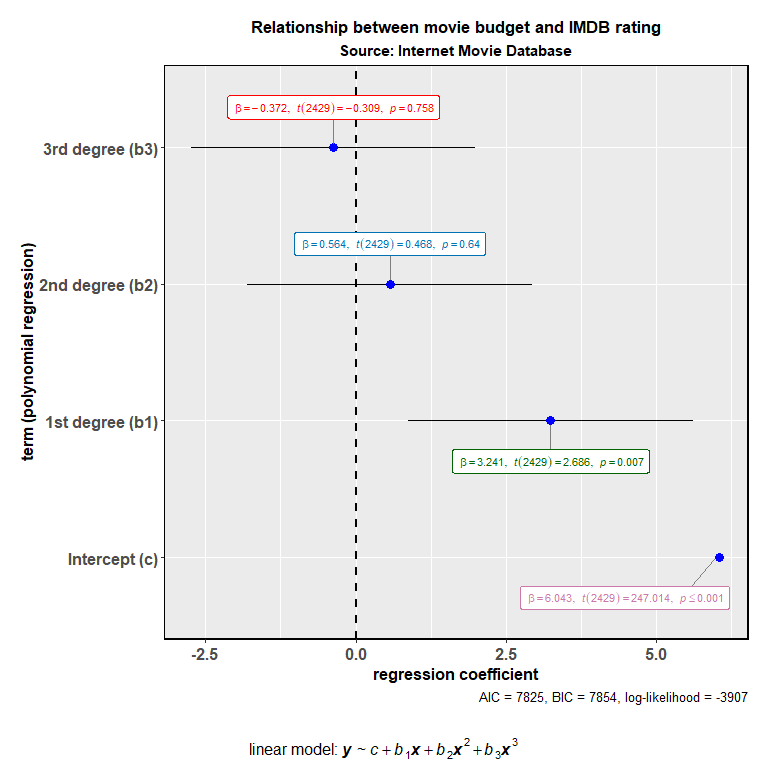# combine_plots

## Combining plots with combine_plots

ggstatsplot also contains a helper function combine_plots to combine multiple plots. This is a wrapper around and lets you combine multiple plots and add combination of title, caption, and annotation texts with suitable default parameters. This is also the

Note before: The codes below illustrates the utility of combine_plots for combining multiple plots created with ggstatsplot functions. But, if you have just one grouping variable, it’s not even necessary to use purrr or plyr since the grouped_ variants (https://indrajeetpatil.github.io/ggstatsplot/reference/index.html) of all functions will take care of this for you and rely on the combine_plots function behind the curtains.

## Combining plots: Examples with purrr

The full power of ggstatsplot can be leveraged with a functional programming package like purrr that replaces many for loops with code that is both more succinct and easier to read and, therefore, purrr should be preferrred.

An example is provided below.

library(glue)
library(dplyr)
library(ggplot2)

### creating a list column with ggstatsplot plots
plots <- datasets::iris %>%
dplyr::mutate(.data = ., Species2 = Species) %>% # just creates a copy of this variable
dplyr::group_by(.data = ., Species) %>%
tidyr::nest(data = .) %>%                        # creates a nested dataframe with list column called data
dplyr::mutate(                                   # creating a new list column of ggstatsplot outputs
.data = .,
plot = data %>%
purrr::map(
.x = .,
.f = ~ ggstatsplot::ggscatterstats(
data = .,
x = Sepal.Length,
y = Sepal.Width,
messages = FALSE,                        # turns off all the warnings, notes, and reference messages
marginal.type = "boxplot",
title =
glue::glue("Species: {.$Species2} (n = {length(.$Sepal.Length)})")
)
)
)

### display the new object (notice that the class of the plot list column is S3: gg)
plots
#> # A tibble: 3 x 3
#>   Species    data              plot
#>   <fct>      <list>            <list>
#> 1 setosa     <tibble [50 x 5]> <S3: ggExtraPlot>
#> 2 versicolor <tibble [50 x 5]> <S3: ggExtraPlot>
#> 3 virginica  <tibble [50 x 5]> <S3: ggExtraPlot>

### creating a grid with cowplot
ggstatsplot::combine_plots(
plotlist = plots$plot, # list column containing all ggstatsplot objects labels = c("(a)", "(b)", "(c)"), nrow = 3, ncol = 1, title.text = "Relationship between sepal length and width for all Iris species", title.size = 14, caption.text = expression( paste( italic("Note"), ": Iris flower dataset was collected by Edgar Anderson.", sep = "" ), caption.size = 12 ) )Here is another example with ggbetweenstats- library(dplyr) library(glue) ### creating a list column with ggstatsplot plots plots <- datasets::mtcars %>% dplyr::mutate(.data = ., cyl2 = cyl) %>% # just creates a copy of this variable dplyr::group_by(.data = ., cyl) %>% # tidyr::nest(data = .) %>% # creates a nested dataframe with list column called data dplyr::mutate( # creating a new list column of ggstatsplot outputs .data = ., plot = data %>% purrr::map( .x = ., .f = ~ ggstatsplot::ggbetweenstats( data = ., x = am, y = mpg, plot.type = "box", # type of plot needed messages = FALSE, # turns off all the warnings, notes, and reference messages xlab = "Transmission", ylab = "Miles/(US) gallon", title = glue::glue( "Number of cylinders: {.$cyl2}"       # this is where the duplicated cyl2 column is useful
)
)
)
)

### display the new object (notice that the class of the plot list column is S3: gg)
plots
#> # A tibble: 3 x 3
#>     cyl data               plot
#>   <dbl> <list>             <list>
#> 1     6 <tibble [7 x 11]>  <S3: gg>
#> 2     4 <tibble [11 x 11]> <S3: gg>
#> 3     8 <tibble [14 x 11]> <S3: gg>

### creating a grid with cowplot
ggstatsplot::combine_plots(
plotlist = plots$plot, # list column containing all ggstatsplot objects nrow = 3, ncol = 1, labels = c("(a)","(b)","(c)"), title.text = "MPG and car transmission relationship (for each cylinder count)", title.size = 13, title.color = "blue", caption.text = expression( paste( italic("Transmission"), ": 0 = automatic, 1 = manual", sep = "" ), caption.size = 10, caption.color = "red" ) )## Combining plots: Examples with plyr Another popular package for handling big datasets is plyr, where we can repeatedly apply a common function on smaller pieces and then combine the results. We’ll use the ggpiestats function as an example function and the movies_long dataset from ggstatsplot package. This dataset provides information about movies scraped from IMDB. Let’s say we are interested in figuring out whether the proportion of different types of MPAA ratings a given genre gets differs across genres (for example, Action movie are more likely to get R-rating, while animated movies to get PG rating?). library(plyr) library(ggstatsplot) # for reproducibility set.seed(123) # let's have a look at the structure of the data dplyr::glimpse(x = ggstatsplot::movies_long) #> Observations: 2,433 #> Variables: 8 #>$ title  <fct> Shawshank Redemption, The, Lord of the Rings: The Retur...
#> $year <int> 1994, 2003, 2001, 2002, 1994, 1993, 1977, 1980, 1968, 2... #>$ length <int> 142, 251, 208, 223, 168, 195, 125, 129, 158, 135, 93, 1...
#> $budget <dbl> 25.0, 94.0, 93.0, 94.0, 8.0, 25.0, 11.0, 18.0, 5.0, 3.3... #>$ rating <dbl> 9.1, 9.0, 8.8, 8.8, 8.8, 8.8, 8.8, 8.8, 8.7, 8.7, 8.7, ...
#> $votes <int> 149494, 103631, 157608, 114797, 132745, 97667, 134640, ... #>$ mpaa   <fct> R, PG-13, PG-13, PG-13, R, R, PG, PG, PG-13, R, PG, R, ...
#> $genre <fct> Drama, Action, Action, Action, Drama, Drama, Action, Ac... # creating a list of plots plots <- plyr::dlply( .data = ggstatsplot::movies_long, .variables = .(genre), .fun = function(data) ggstatsplot::ggpiestats( data = data, main = mpaa, title = glue::glue("Genre: {data$genre}")
)
)

# combining individual plots
ggstatsplot::combine_plots(plotlist = plots,
title.text = "Differences in MPAA ratings by movie genre",
title.color = "darkgreen",
caption.text = "MPAA: Motion Picture Association of America",
caption.color = "orange",
nrow = 3,
ncol = 2)As can be seen from these plots, our hunch was correct: all ratings are not equally likely for any of the genres, for example Dramas and Documentary genre movies most frequently R-rating, while Animations genre movies overwhelmingly get PG rating.

## Combining plot with additional text

This function can also be useful adding some additional text information that can’t be contained in either title, subtitle, or caption. Here is an example: We want to run a polynomial regression and want to annotate the formula.

library(ggstatsplot)

ggstatsplot::combine_plots(
# preparing the plot with ggstatsplot function
ggstatsplot::ggcoefstats(
x = stats::lm(
formula = rating ~ stats::poly(budget, degree = 3),
data = ggstatsplot::movies_long,
na.action = na.omit
),
exclude.intercept = FALSE,
title = "Relationship between movie budget and IMDB rating",
subtitle = "Source: Internet Movie Database",
ggtheme = ggplot2::theme_gray(),
stats.label.color = c("#CC79A7", "darkgreen", "#0072B2", "red")
) +
# modifying the plot outside of ggstatsplot using ggplot2 functions
ggplot2::scale_y_discrete(
labels = c(
"Intercept (c)",
"1st degree (b1)",
"2nd degree (b2)",
"3rd degree (b3)"
)
) +
ggplot2::labs(y = "term (polynomial regression)"),
sub.text = expression(
paste(
"linear model: ", bolditalic(y),
" ~ ",
italic(c) + italic(b) * bolditalic(x) + italic(b) * bolditalic(x) ^
2 + italic(b) * bolditalic(x) ^ 3,
sep = ""
)
),
sub.size = 12
)## Suggestions

If you find any bugs or have any suggestions/remarks, please file an issue on GitHub: https://github.com/IndrajeetPatil/ggstatsplot/issues